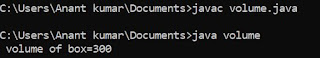## Wednesday, 11 July 2018

### Multiplication of matrix of order 2 * 2 in C programming language

/* multiplication of matrix */
# include <stdio.h>
void main ()
{
int a {2}{2} , b {2}{2} , c {2}{2} ;
int i , j ;
clrscr () ;
for ( i = 0 ; i < 2 ; i++ )                   /* Input of first matrix */
{
for ( j = 0 ; j < 2 ; j++ )
{
printf ("\n Enter a number:") ;
scanf ("%d", & a {i}{j}) ;
}
}
for ( i = 0 ; i < 2 ; i++ )                    /* Input of second matrix */
{
for ( j = 0 ; j < 2 ; j++ )
{
printf ("\n Enter a number:") ;
scanf ("%d", & b {i}{j}) ;
}
}

for ( i = 0 ; i < 2 ; i++ )             /* print first matrix */
{
for ( j = 0 ; j < 2 ; j++ )
printf ("%3 d", a {i}{j}) ;
printf ("\n") ;
}
for ( i = 0 ; i < 2 ; i++ )              /* print second matrix */
{
for ( j = 0 ; j < 2 ; j++ )
printf ("%3 d", b{i}{j}) ;
printf ("\n") ;
}
for ( i = 0 ; i < 2 ; i++ )
{
for ( i = 0 ; i < 2 ; i++ )
{
c [i][j] = 0 ;
for ( k = 0 ; k < 2 ; k++ )
{
c [i][j] = c [i][j] + a [i][k] * b [k][j] ;        /* calculation of multiplication */
}
}
}
for ( i = o ; i < 2 ; i++ )
{
for ( j = 0 : j < 2 ; j++ )
{
printf ("% 3d", c [i][j]) ;
printf ("\n") ;
}
}
getch () ;
}

Here we write program to calculate the multiplication of matrix of order 2 * 2 in C . Here we input the matrix from the user which is must contain 2 rows and 2 columns . In C programming we use 2 - D array . As we know that multiplication of matrix is possible only when number of rows of first matrix is equal to number of column of second matrix but here we have not to check because no of rows and column is fixed for both matrix .